# Learn Embedded Programming [Step by Step]

## Embedded Programming Tutorials for Beginners with Step By Step Guide.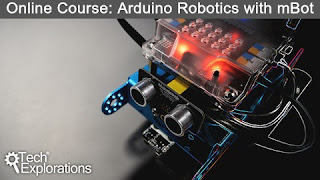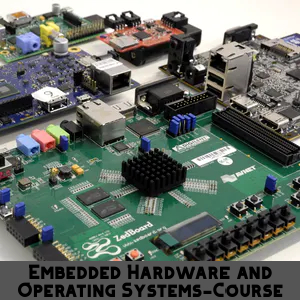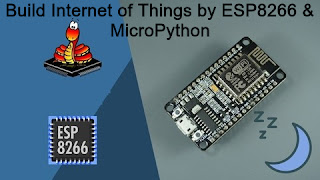## IR Controlled LED Switching : 5th & LAST PART

I have to make it quick and I think we have got all required information. I ain't going to describe source code. You have to help yourself. It has become already too long.
I can honestly say that I tried my best. Today I am going to finish this series and it's the last part.

So first of all, take a look at calculation part.

## Final Calculation :

To identify each bit uniquely, we will use another calculation method. You may use as you want. I ain't going to use millisecond. You can use millisecond or microsecond if you want.

If you want to use millisecond to measure pulse, then for logical 0 you get (562us_on+562us_off)=1.124ms. and for logical 1 you get (562us_on+1687us_off)=2.249ms.

You know, for each task  microcontroller needs time. That's why reading can be varied from calculation. So if you use integer number, you will get 1ms for logical 0 and 2ms for 1. By applying this you may successfully able to make it. Alright.

#### Now I am going to show about my method that was used on my project:

we know that , 1secon means=750000 pulses. Alright.
So, 1000ms means=750000 pulses and 1000000us means=750000 pulses.

Now, 1000000us means=750000 pulse
and   1us     ,,  = (750000/1000000)=0.75 pulses
and  562us   ,,   =(0.75*562)=421.5=421 pulses

Again,
So, 1000000us means=750000 pulse
and   1us     ,,  = (750000/1000000)=0.75 pulses
and  1687us   ,,   =(0.75*1687)=1265.25=1265 pulses

#### So, for logical 1 :

(562+1687) or (421.5+1265.75)=1686 pulses

Now, we know 255 pulses means 1 interrupt(timer overflow) or Count=1.
So ,                      1    ,,     ,,    (1/255)
So ,                    1686   ,,     ,,   (1686/255)=6.62

So, we can say 6.62 interrupts means logical 1. In coding we I will use 5 or 6 or 7 to identify logical 1 in proteus.

### So, for logical 0 :

(562+562) or (421.5+421.5)=843 pulses

Now, we know 255 pulses means 1 interrupt
(timer overflow) or Count=1.
So ,          1    ,,     ,,  (1/255) or count=(1/255)
so ,        843   ,,     ,,   (843/255)=3.31 or count=3

So, we can say 3.31 interrupts means logical 0. In coding we I will use 2 or 3 or 4 to identify logical 0 in proteus.
By using this technique, from count variable we can identify logical 1 or logical 0.

## ***Transmitter Section(Important):

In source code of transmitter , 4 commands are used .

#define command_2 0x81 or 10000001
#define command_3 0x42 or 01000010
#define command_4 0xC3 or 11000011
#define command_5 0x24 or 00100100

Here blue color represents 4bit command and red color represents reverse order of the main 4bit command (blue colored).To avoid noise and to achieve clear signal we need this.

Consider that we have main 4bit command 0001.So the reverse command should be 1000 command .Now we have to attach these and that will be 10000001. Finally we get 8bit command in which a main command and a reverse of that command are saved .

### Now We need some knowledge about PWM(Pulse Width Modulation)

We will use PWM to generate 36KHz pulse and that's why we will connect positive(+) pin of Transmitter unit  Microcontroller's  CPP1 pin . Because using that pin of Microcontroller will generate 36KHz pulse .

PWM1_Init(36000); will set up the CPP1 pin for PWM .

PWM1_Set_Duty(127);

set_duty(127) is used to providing same for both ON and OFF .That means (T/2) time it will ON and (T/2) time it will OFF .If we use set_duty(255) , then total Time period (T) will be remain ON. If we use set_duty(0) , then total Time period (T) will be remain OFF.IR (Infrared) Remote Controlled Communication Between Two Microcontroller -Step By Step Tutorial : LAST PART ( END )IR (Infrared) Remote Controlled Communication Between Two Microcontroller -Step By Step Tutorial : LAST PART ( END )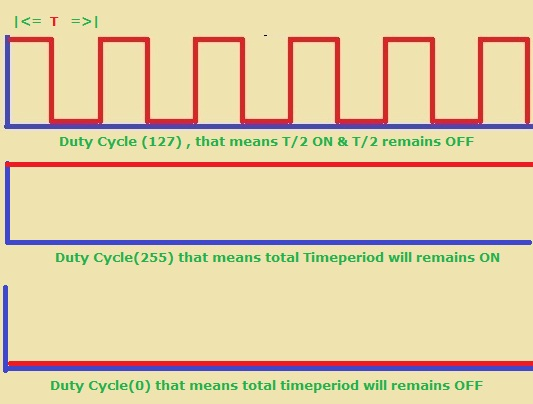IR (Infrared) Remote Controlled Communication Between Two Microcontroller -Step By Step Tutorial : LAST PART ( END )

### Again look at another picture given below

Here in if statement , an AND operation performed between Command and 0x01 . We are using this to identify each bit of data command.
Suppose , our command is 10000001 and 0x01=00000001.So ,when an AND operation performs the result will be like this.Shifting Operation in ProgrammingShifting Operation in Programming

#### So, if result is 0 ,then the related bit is 0 and delay should be 562us.

Here this part of code is comparing that Data signal commands received in receiver unit are correct .

``````   if(val==val){
txt=val;
}
if(val==val){
txt=val;
}
if(val==val){
txt=val;
}
if(val==val){
txt=val;
}
``````

## ``````  if(txt=='0' && txt=='0' && txt=='0' && txt=='1') { PORTB.F6=1; PORTB.F5=0; PORTB.F4=0; PORTB.F3=0; } ``````

When microcontroller understands about the 4bit command "0001" , consequently it turns ON RB6 pin or First LED .

## Transmitter:

``````

#define command_1 0xFF
#define command_2 0x81
#define command_3 0x42
#define command_4 0xC3
#define command_5 0x24
unsigned int i=0,j=0;
void repeat(){
PWM1_Start();
delay_us(8000);
PWM1_Stop();
delay_us(8000);
}
send_data_byte(unsigned char dattt){
PWM1_init(36000);
PWM1_Set_Duty(127);
for(j=0;j<8;j++){
PWM1_Start();
delay_us(562);
PWM1_Stop();
if(dattt & 0x01){
delay_us(1687);
dattt=dattt>>1;
}
else
{
delay_us(562);
dattt=dattt>>1;
}
}
PWM1_Stop();
}
void send_data_command(unsigned char dat){
PWM1_init(36000);
PWM1_Set_Duty(127);
PWM1_Start();
delay_us(9000);
PWM1_Stop();
delay_us(4500);
send_data_byte(dat);
repeat();
}
void main() {
CMCON=7; // to disable comparator
TRISA.F0=1;
TRISA.F1=1;
TRISA.F2=1;
TRISA.F3=1;
TRISC.F2=0;

PORTC.F2=0;
while(1){
if(PORTA.F0==0){
send_data_command(command_2);
while(PORTA.F0==0){
repeat();
}
}
if(PORTA.F1==0){
send_data_command(command_3);
while(PORTA.F1==0){
repeat();
}
}
if(PORTA.F2==0){
send_data_command(command_4);
while(PORTA.F2==0){
repeat();
}
}
if(PORTA.F3==0){
send_data_command(command_5);
while(PORTA.F3==0){
repeat();
}
}
}
}

``````

``````

unsigned int count=0,sgnal=0;
int i=7,j=0,xr=0;
char txt[]="........";
char val[]="........";
void timer_routin(){
count++;
if(count>0xFF){
count=0;
TMR0L=0;
}
}

void interrupt()
{
if(INTCON.TMR0IF){
timer_routin();
INTCON.TMR0IF=0;
}
if(INTCON.INT0IF){

xr=count;
count=0;
TMR0L=0;
if(i<0){ i=7; }
if(xr==3){ // you may use if(xr==3``````||xr==4||xr==2){...}
val[i]='0';
i--;
if(i<0){ i=7; }
}
else if(xr==6){  ``````// you may use if(xr==5||xr==6||xr==7){...}
val[i]='1';
i--;
if(i<0){ i=7; }
}
else{
/// For start signal or Repeat signal , i=0 and start to count
TMR0L=0;
i=7;
count=0;
}
INTCON.INT0IF=0;
}
}
void main()
{
CMCON=7;
TRISB=0x01;
PORTB.F6=0;
PORTB.F5=0;
PORTB.F4=0;
PORTB.F3=0;
T0CON=0xC1;
TMR0L=0;
INTCON.GIE=1;
INTCON.PEIE=1;
INTCON.TMR0IE=1;
INTCON.INT0IE=1;
INTCON.TMR0IF=0;
INTCON.INT0IF=0;
INTCON2.RBPU=1; // DISABLE PULUP REGISTER
INTCON2.INTEDG0=0;
while(1){
if(val==val){
txt=val;
}
if(val==val){
txt=val;
}
if(val==val){
txt=val;
}
if(val==val){
txt=val;
}
if(txt=='0' && txt=='0' && txt=='0' && txt=='1') {
PORTB.F6=1;
PORTB.F5=0;
PORTB.F4=0;
PORTB.F3=0;
}
if(txt=='0' && txt=='0' && txt=='1' && txt=='0') {
PORTB.F6=0;
PORTB.F5=1;
PORTB.F4=0;
PORTB.F3=0;
}
if(txt=='0' && txt=='0' && txt=='1' && txt=='1') {
PORTB.F6=0;
PORTB.F5=0;
PORTB.F4=1;
PORTB.F3=0;
}
if(txt=='0' && txt=='1' && txt=='0' && txt=='0') {
PORTB.F6=0;
PORTB.F5=0;
PORTB.F4=0;
PORTB.F3=1;
}
}
}

``````

## Watch Video for Proteus 8

Watch Video in Real Life :

### Thank You!

1.1.Thank you too.

2.Many thanks for the Great tutorial and Valuable files

1.Thank you too.

3.how do i make a pin for speed control as dimmer using triac for ac appliances.

1.Yes you can. This case you need to use PWM pin connected with the gate. If you increase the PWM width the AC current will follow through the triac. I have no practical experience on that case. I will suggest you not to use DIAC between the pin and gate since as far as I know DIAC needs probably bit high voltage to make conduction and Microcontroller pin will provide 3.3 volts or 5 volts(You shlould check through a multimeter to measure pin voltage). Best of luck. It's a late reply since my comment option wasn't working. Suddenly I've found it's working. Anyway thank you for your comment.

4.Can you explain tihs part?
PWM1_init(36000);
PWM1_Set_Duty(127);
PWM1_Start();

1.1.PWM1_init(36000); means initializing PWM1 with that frequency.
2. PWM1_Set_Duty(127); making duty cycle 1/2. That means on & have same time duration.
3.PWM1_Start(); starting the PWM1 at the certain pin.

5.Sir I want circuit diagram with name in Proteus so that I can simulate in Proteus my mail I'd is rpandey8800 @gmail.com if u sent the pic of Proteus connect with component name in Proteus I will very thankful to you

1.Download option was given up there at the bottom of the post.

6.The mortgage calculator is described as being Canadian, and also by default, it will calculate a Canadian mortgage, however it also gets the ability to calculate US mortgages. mortgage payment calculator In Canada you can find similar concepts to DTI the location where the Canada Mortage and Housing Corporation (CMHC) sets limits to shield homeowners from loans they are unlikely to afford paying. canadian mortgage calculator

7.I was more than happy to uncover this great site. I need to thank you for your time due to this fantastic read!!
I definitely enjoyed every bit of it and I have you bookmarked to see new information on your blog.
humidifier dehumidifier combo

8.I was more than happy to uncover this great site. I need to thank you for your time due to this fantastic read!!
I definitely enjoyed every bit of it and I have you bookmarked to see new information on your blog.
WordPress Chat Plugin

9.Pretty good post. I just stumbled upon your blog and wanted to say that I have really enjoyed reading your blog posts. Any way I’ll be subscribing to your feed and I hope you post again soon. infrared heating pad for back pain

10.Great knowledge, do anyone mind merely reference back to it ceramic heating element

11.Has anyone been able to find out which is the best product among those listed here?
Best Recumbent Exercise Bike

## Course by The University of Texas at Austin

(FREE Edx Course) Embedded Systems - Shape The World: Microcontroller Input/Output. Offered by The University of Texas## Become a Robotics Software Engineer- Udacity Course

Ain't getting any visitors!

## Featured Post

### Rs 232 , Serial Communication with PIC Microcontroller in Proteus [step by step details]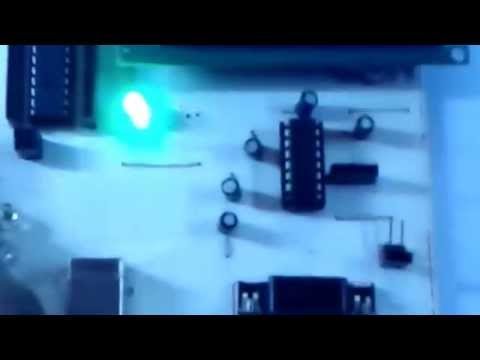## Tags

: (1) 18F2550 (1) 36KHz (3) and (1) arduino (1) Based (1) battery (1) Bipolar (1) Blinking (1) blinks (1) Bluetooth (1) button (1) circuit (1) clock (1) control (1) Db9 (1) DC Motor (2) digital (2) display (2) DS1307 (1) electronic (1) flash (1) flashing (1) HC-06 (1) home (1) how (1) How to (10) i2c tutorial (1) in (1) indicator (1) interface (8) interfacing (3) Interrupt (3) Introduction (1) IR Receiver (4) key pad (1) keyboard (1) keypad (1) lavel (1) Lcd 16x2 (2) lcd 2x16 (2) led (1) lm35 (2) LPG (1) machine (1) make (1) making (1) matrix (1) max232 (1) meter (2) microchip (4) microchips (3) mikroC (5) musical (1) NEC Protocol (4) pcb (5) PIC (3) pic controller (11) pic proteus (1) Pic Tutorial (12) pic18 (2) pic18f2550 (11) picRFモジュール (1) proteus (6) push (1) push button (1) PWM (1) real (1) Rs 232 (1) Rs232 (1) scroll (1) scrolling (1) Serial Port (1) simulation (2) step by step (7) step bystep (1) text (2) time (1) timer (4) timer0 (4) tone (1) tutorial (2) Unipolar (1) USB (1) usb 1.0 (1) USB HID (1) using (9) voltmeter (1) voting (1) with (2) work (1)

## Traffic Feed

Live Traffic Feed
Visitor Tracking